# Samacheer Kalvi 12th Maths Solutions Chapter 8 Differentials and Partial Derivatives Ex 8.8

## Tamilnadu Samacheer Kalvi 12th Maths Solutions Chapter 8 Differentials and Partial Derivatives Ex 8.8

Choose the correct or the most suitable answer from the given four alternatives.

Question 1.
A circular template has a radius of 10 cm. The measurement of radius has an approximate error of 0.02 cm. Then the percentage error in calculating area of this template is …….
(a) 0.2%
(b) 0.4%
(c) 0.04%
(d) 0.08%
Solution:
(b) 0.4%
Hint:
r = 10 cm
dr = 0.02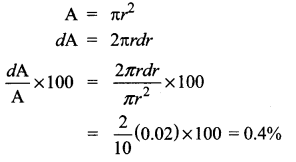Question 2.
The percentage error of fifth root of 31 is approximately how many times the percentage error in 31?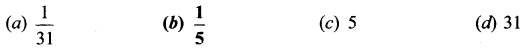Solution:
$$\frac{1}{5}$$
Hint:
We know that the percentage error in the «th root of a number is approximately $$\frac{1}{n}$$ times the percentage error in the number.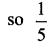Question 3.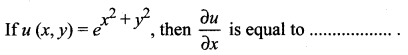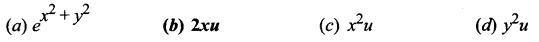Solution:
(b) 2xu
Hint: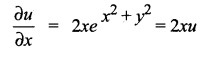Question 4.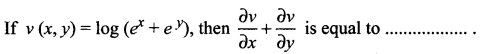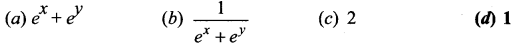Solution:
(d) 1
Hint: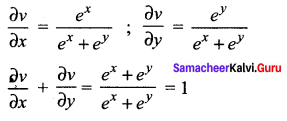Question 5.
If w (x, y) = xy, x > 0, then $$\frac{\partial w}{\partial x}$$ is equal to ……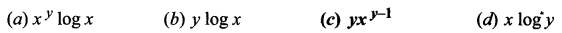Solution:
(c) yxy – 1
Hint: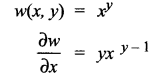Question 6.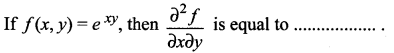(a) xyexy
(b) (1 + xy)exy
(c) (1 + y)exy
(d) (1 + x)exy
Solution:
(b) (1 + xy)exy
Hint: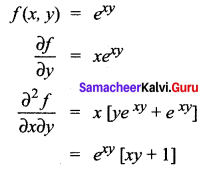Question 7.
If we measure the side of a cube to be 4 cm with an error of 0.1 cm, then the error in our calculation of the volume is …….
(a) 0.4 cu.cm
(b) 0.45 cu.cm
(c) 2 cu.cm
(d) 4.8 cu.cm
Solution:
(d) 4.8 cu.cm
Hint.
a = 4 cm
da = 0.1 cm
v = a3
dv = 3a2da
= 3(4)2 (0.1)
= 4.8 cu. cmQuestion 8.
The change in the surface area S = 6x2 of a cube when the edge length varies from x0 to x0 + dx is …….
(a) 12x0 + dx
(b) 12x0dx
(c) 6x0dx
(d) 6x0 + dx
Solution:
(b) 12x0dx

Question 9.
the approximate change in the volume: V of a cube of side x mentres caused by increasing the side by 1% is ……..
(a) 0.3 xdx m3
(b) 0.03 x m3
(c) 0.03 x2 m3
(d) 0.03 x3m3
Solution:
(d) 0.03 x3m3
Hint:
Let the side of the cube be x units
v = x3
when dx = 0.01x
dv = 3x2dx
= 3x2(0.01 x)
= 0.03 x3m3

Question 10.
If g(x, y) = 3x2 – 5y + 2y2, x(t) = et and y(t) = cos t, then $$\frac{d g}{d t}$$ is equal to ……..
(a) 6e2t + 5 sin t – 4 cos t sin t
(b) 6e2t – 5 sin t + 4 cos t sin t
(c) 3e2t + 5 sin t + 4 cos t sin t
(d) 3e2t – 5 sin t + 4 cos t sin t
Solution:
(a) 6e2t + 5 sin t – 4 cos t sin t

Question 11.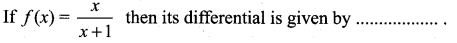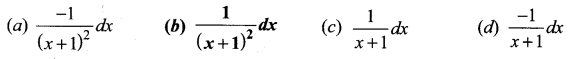Solution:
(b) $$\frac{1}{(x+1)^{2}} d x$$
Hint: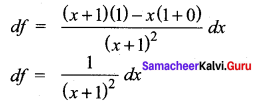Question 12.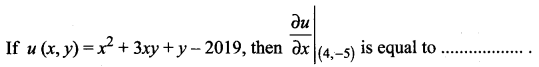(a) -4
(b) -3
(c) -7
(d) 13
Solution:
(c) -7
Hint: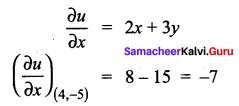Question 13.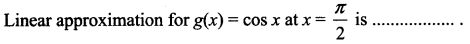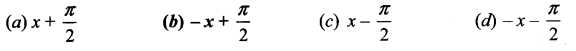Solution:
(b) $$-x+\frac{\pi}{2}$$
Hint: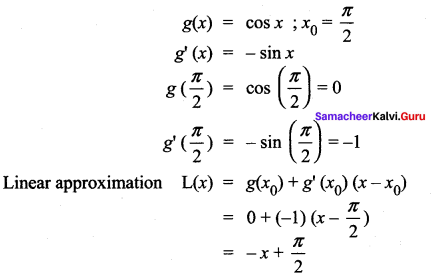Question 14.
If w(x, y, z) = x2 (y – z) + y2 (z – x) + z2 (x – y), then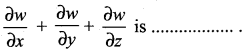(a) xy + yz + zx
(a) xy + yz + zx
(b) x(y + z)
(c) y(z + x)
(d) 0
Solution:
(d) 0
Hint: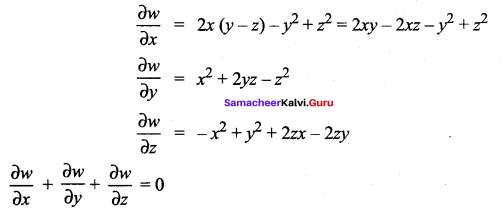Question 15.
If f(x, y, z) = xy + yz + zx, then fx – fz is equal to ……….
(a) z – x
(b) y – z
(c) x – z
(d) y – x
Solution:
(a) z – x
Hint:
fx = y + z
fz = y + x
fx – fz = y + z – y – x = z – x

Question 1.
If u = xy then is equal to ……..
(a) yxy – 1
(b) u log x
(c) u log y
(d) xyx – 1
Solution:
(a) yxy – 1
Hint: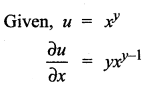Question 2.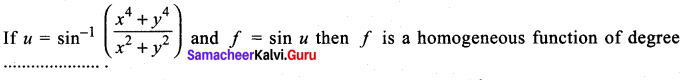(a) 0
(b) 1
(c) 2
(d) 4
Solution:
(c) 2
Hint: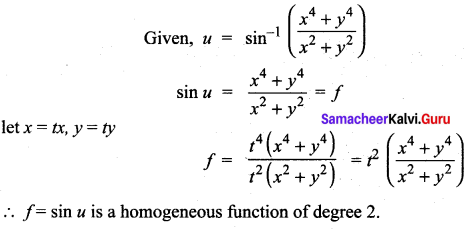Question 3.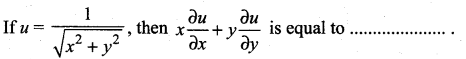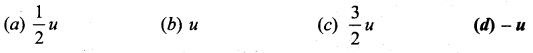Solution:
(d) -u
Hint: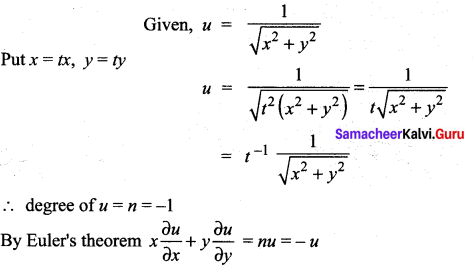Question 4.
The curve y2 (x- 2) = x2 (1 +x) has …….
(a) an asymptote parallel to x-axis
(b) an asymptote parallel to y-axis
(c) asymptotes parallel to both axis
(d) no asymptotes
Solution:
(b) an asymptote parallel to y-axis
Hint: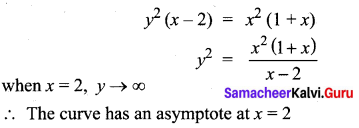Question 5.
If x = r cos θ, y = r sin θ, then $$\frac{\partial r}{\partial x}$$ is equal to …….
(a) sec θ
(b) sin θ
(c) cos θ
(d) cosec θ
Solution:
(c) cos θ
Hint: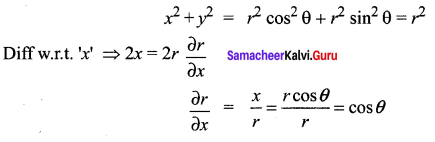Question 6.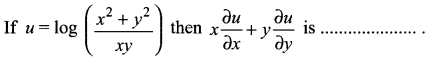(a) 0
(b) u
(c) 2u
(d) u – 1
Solution:
(a) 0
Hint: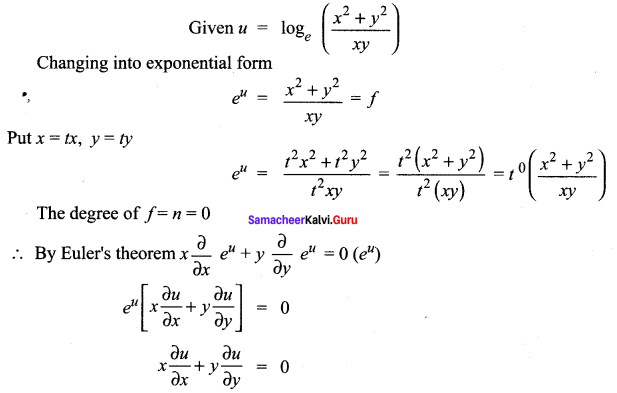Question 7.
The percentage error in the 11th root of the number 28 is approximately …. times the precentage error is 28.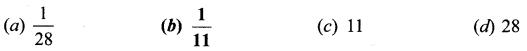Solution:
$$\frac{1}{11}$$
Hint: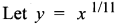Take log on both sides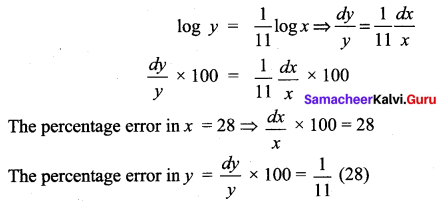The percentage error is approximately $$\frac{1}{11}$$ times the percentage error is 28.

Question 8.
The curve a2y2 = x2 (a2 – x2) has ……
(a) only one loop between x = 0 and x = a
(b) two loops between x = 0 and x = a
(c) two loops between x = – a and x = a
(d) no loop
Solution:
(c) two loops between x = – a and x = a
Hint.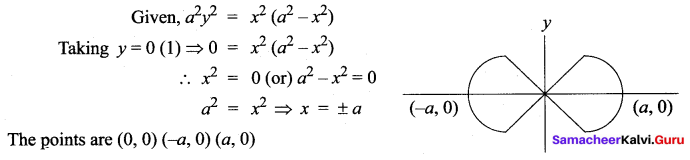Question 9.
An asymptote to the curve y2 (a + 2x) = x2 (3a – x) is ………
(a) x = 3a
(b) x = – a/2
(c) x = a/2
(d) x = 0
Solution:
(b) x = – a/2
Hint.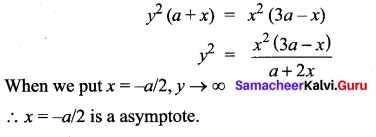Question 10.
In which region the curve y2 (a + x) = x2 (3a – x) does not lie?
(a) x > 0
(b) 0 < x < 3a (c) x ≤ – a and x > 3a
(d) – a < x < 3a Solution: (c) x ≤ – a and x > 3a
Hint.
y2 (a + x) = x2 (3a – x)
Taking y = 0 (1) ⇒ 0 = x2 (3a – x)
= x = 0, x = 3a
∴ The points are (0, 0) (3a, 0)
There is a loop between x = 0 and x = 3a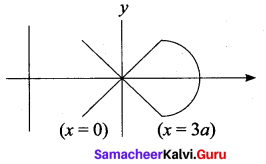when x > 3 a, y ➝ imaginary
the curves does not exist beyond x = 3a i.e., x > 3a
the curve has asymptote at x = -a
the curve does not exist when x < -a
the curve exists in the region – a < x < 3a

Question 11.
If M = y sin x, then $$\frac{\partial^{2} u}{\partial x \partial y}$$ is equal to …….
(a) cos x
(b) cos y
(c) sin x
(d) 0
Solution:
(a) cos x
Hint: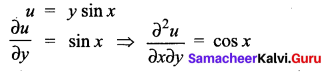Question 12.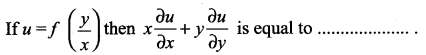(a) 0
(b) 1
(c) 2u
(d) u
Solution:
(a) 0
Hint: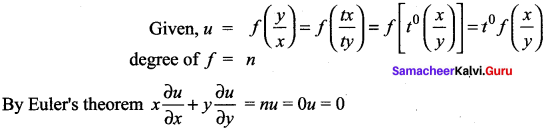Question 13.
The curve 9y2 = x2 (4 – x2) is symmetrical about …….
(a) y – axis
(b) x – axis
(c) y = x
(d) both the axes
Solution:
(d) both the axes
Hint.
Replace x by – x and y by -y
9 (-y2) = (-x)2(4-(-x)2)
The equation is unaltered
∴ the curve is symmetrical about both the axes.Question 14.
The curve ay2 = x2 (3a – x) cuts the y – axis at …….
(a) x = – 3a, x = 0
(b) x = 0, x = 3a
(c) x = 0, x = a
(d) x = 0
Solution:
(d) x = 0
Hint:
Given ay2 = x2 (3a – x)
The point of intersection with y-axis by putting x = O
In (1) = ay2 = 0 (3a – 0)
ay = 0 ; y = 0
∴ The curve intersects y-axis at the origin is x = 0